# Add, Subtract and Simplify Rational Expressions Questions With Detailed Solutions

Detailed solutions to questions in How to Add, Subtract and Simplify Rational Expressions are presented.

## Add, Subtract and Simplify the Following Rational Expressions - Solutions

### Question 1Solution:
The three denominators in the fractions above are different and therefore we need to find a common denominator.
We first find the lowest common multiple (LCM) of the two denominators 6, 18 and 24.
6: 6, 12, 18, 24, 30, 36, 42, 48, 54, 60, 66, 72, 80,...
18: 18, 36, 54, 72, 90,...
24: 24, 48, 72, 96...
The lowest common denominator is 72 and we now convert all 3 denominators to the common denominator 72and simplify as follows: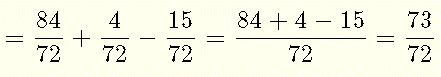### Question 2Solution:
The two rational expressions have different denominators. In order to add the rational expressions above, we need to convert them to a common denominator. The two denominators x + 5 and x + 2 have no common factors hence their LCM is given by:
LCM = (x + 5)(x + 2)
We now use the LCM as the common denominator and rewrite the rational expressions with the same denominator as follows.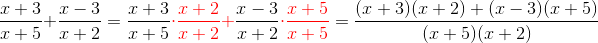We now expand, simplify and factor the numerator if possible .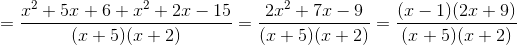### Question 3Solution:
In order to add a rational expression with an expression without denominator, we convert the one without denominator into a rational expression then add them.The two rational expressions have the same denominator and they are added as follows:### Question 4Solution:
The two rational expressions have different denominators. In order to add the rational expressions above, we need to convert them to a common denominator. We first factor completely the two denominators x 2 - 3x + 2 and x 2 + 2 x - 3 and find the LCM of Expressions.
x 2 - 3x + 2 = (x - 1) (x - 2)
x 2 + 2 x - 3 = (x - 1)(x + 3)
LCM = (x - 1)(x - 2)(x + 3)
We now use the LCM as the common denominator and rewrite the rational expressions with the same denominator as follows.We now add the numerators expand and simplify.### Question 5Solution:
We rewrite the given expression with numerators and denominators in factored form and simplify if possible.We cancel common factors.The two denominators x + 1 and x + 3 have no common factors and therefore their LCD is (x + 1)(x + 3). We rewrite the above with the common factor (x + 1)(x + 3) as follows:Expand and simplify.### Question 6Solution:
The three rational expressions have different denominators. In order to subtract/add the rational expressions above, we need to convert them to a common denominator.List and factor completely the three denominators 2x - 1 , 2 x 2 + 9 x - 5 and 2 x + 10 and find the LCM.
2x - 1 = 2x - 1
2 x 2 + 9 x - 5 = (2x - 1)(x + 5)
2x+10 = 2(x + 5)
LCM = 2(2x - 1)(x + 5)
We now use the LCM as the common denominator and rewrite the rational expressions with the same denominator as follows.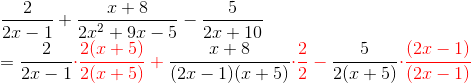We now add the numerators and simplify.### Question 7Solution:
The two rational expressions have different denominators. In order to subtract/add the rational expressions above, we need to convert them to a common denominator. List and factor completely the two denominators y(x y - y + 3 x - 3) and 2 x - 2 and find the LCM.
y(x y - y + 3 x - 3) = y( y(x - 1) + 3 (x - 1)) = y(x - 1)(y + 3)
2 x - 2 = 2(x - 1)
LCM = 2 y (x - 1)(y + 3)
We now use the LCM as the common denominator and rewrite the rational expressions with the same denominator as follows.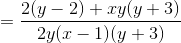Expand and simplify.